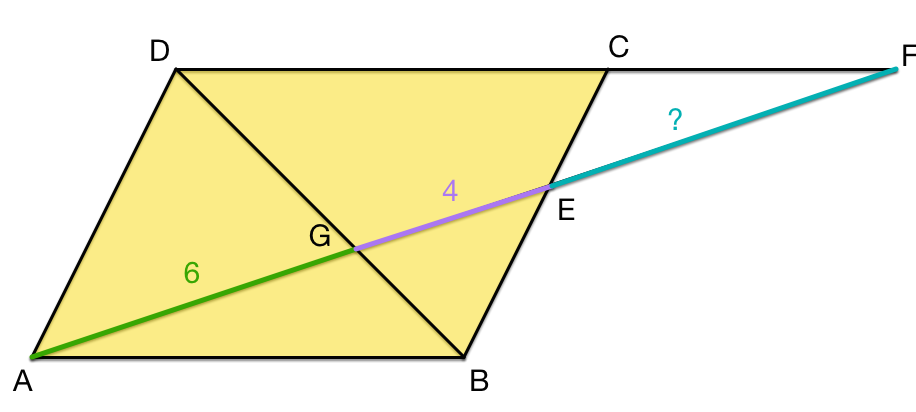# Simple Similarity

Geometry Level 3$ABCD$ is a parallelogram. The point $E$ is in the segment $BC$, and $F$ is a point in the line $DC$ such that $AF$ pass through the point $E$ and intersect the segment $BD$ in the point $G$. If $AG=6$ and $GE=4$. How long is $EF$?

×